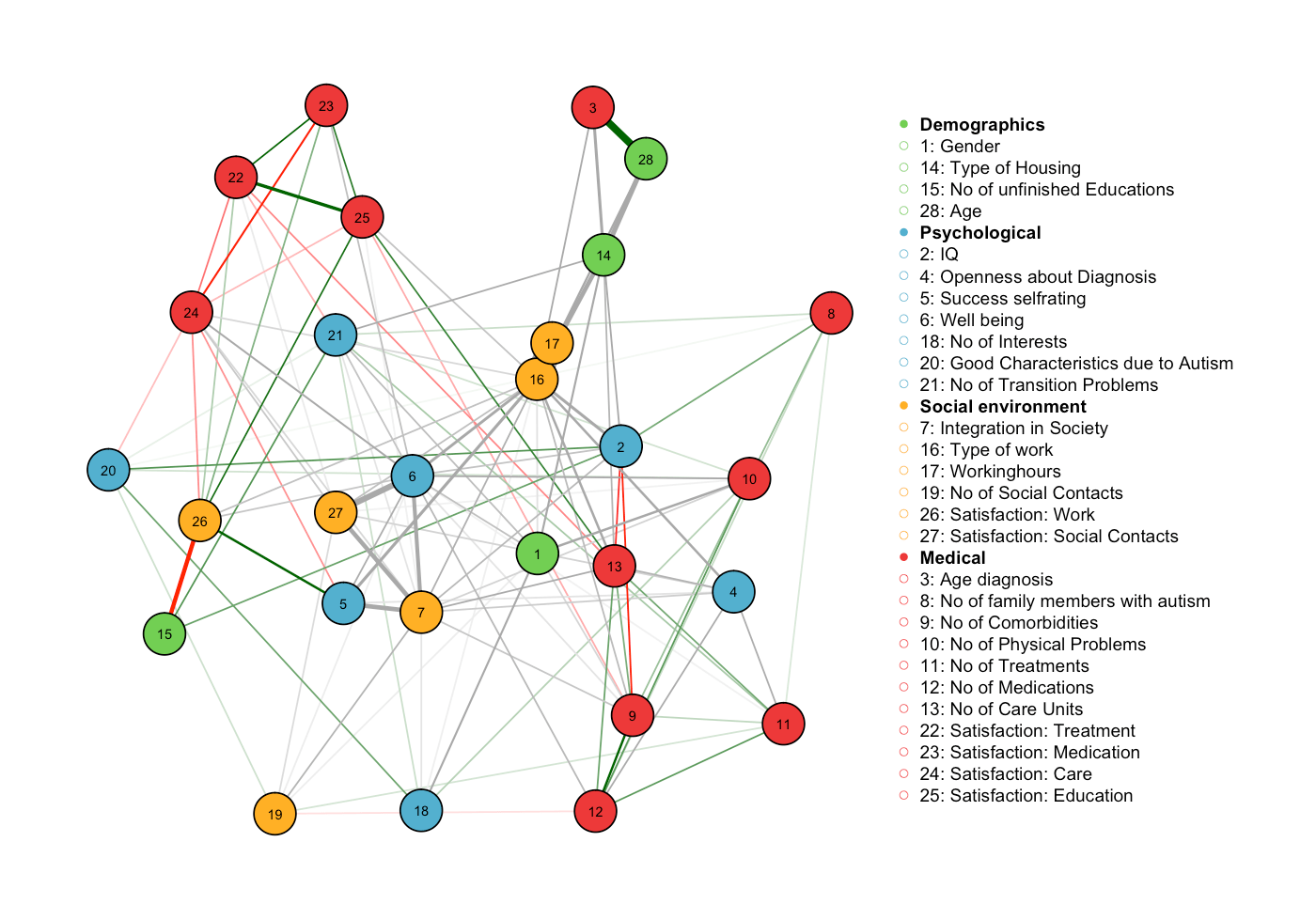Want to share your content on R-bloggers? click here if you have a blog, or here if you don't.

Determining conditional independence relationships through undirected graphical models is a key component in the statistical analysis of complex obervational data in a wide variety of disciplines. In many situations one seeks to estimate the underlying graphical model of a dataset that includes variables of different domains.

As an example, take a typical dataset in the social, behavioral and medical sciences, where one is interested in interactions, for example between gender or country (categorical), frequencies of behaviors or experiences (count) and the dose of a drug (continuous). Other examples are Internet-scale marketing data or high-throughput sequencing data.

There are methods available to estimate mixed graphical models from mixed continuous data, however, these usually have two drawbacks: first, there is a possible information loss due to necessary transformations and second, they cannot incorporate (nominal) categorical variables (for an overview see here). A new method implemented in the R-package mgm addresses these limitations.

In the following, we use the mgm-package to estimate the conditional independence network in a dataset of questionnaire responses of individuals diagnosed with Autism Spectrum Disoder. This dataset includes variables of different domains, such as age (continuous), type of housing (categorical) and number of treatments (count).

The dataset consists of responses of 3521 individuals to a questionnaire including 28 variables of domains continuous, count and categorical.

```dim(data)
##  3521   28

round(data[1:4, 1:5],2)
##      sex IQ agediagnosis opennessdiagwp successself
## [1,]   1  6            0              1        1.92
## [2,]   2  6            7              1        5.40
## [3,]   1  5            4              2        5.66
## [4,]   1  6            8              1        8.00```

We now use our knowledge about the variables to specify the domain (or type) of each variable and the number of categories for categorical variables (for non-categorical variables we choose 1). “c”, “g”, “p” stands for categorical, Gaussian and Poisson (count), respectively.

```type <- c("c", "g", "g", "c", "c", "g", "c", "c", "p", "p",
"p", "p", "p", "p", "c", "p", "c", "g", "p", "p",
"p", "p", "g", "g", "g", "g", "g", "g", "c", "c",
"g")

cat <- c(2, 1, 1, 3, 2, 1, 5, 3, 1, 1, 1, 1, 1, 1, 2, 1, 4,
1, 1, 1, 1, 1, 1, 1, 1, 1, 1, 1, 3, 2, 1)```

The estimation algorithm requires us to make an assumption about the highest order interaction in the true graph. Here we assume that there are at most pairwise interactions in the true graph and set d = 2. The algorithm includes an L1-penalty to obtain a sparse estimate. We can select the regularization parameter lambda using cross validation (CV) or the Extended Bayesian Information Criterion (EBIC). Here, we choose the EBIC, which is known to be a bit more conservative than CV but is computationally faster.

```library(mgm)

fit <- mgmfit(data, type, cat, lamda.sel="EBIC", d=2)```

The fit function returns all estimated parameters and a weighted and unweighted (binarized) adjacency matrix. Here we use the qgraph package to visualize the graph:

```# group variables
group_list <- list("Demographics"=c(1,14,15,28),
"Psychological"=c(2,4,5,6,18,20,21),
"Social environment" = c(7,16,17,19,26,27),
"Medical"=c(3,8,9,10,11,12,13,22,23,24,25))

# define nice colors
group_cols <- c("#E35959","#8FC45A","#4B71B3","#E8ED61")

# plot
library(qgraph)
vsize=3, layout="spring",
edge.color = rgb(33,33,33,100,
maxColorValue = 255),
color=group_cols,
border.width=1.5,
border.color="black",
groups=group_list,
nodeNames=datalist\$colnames,
legend=TRUE,
legend.mode="groups",
legend.cex=.75)```A reproducible example can be found in the examples of the package or more elaboratly explained in the corresponding paper. Here is a paper explaining the theory behind the implemented algorithm.

Computationally efficient methods for Gaussian data are implemented in the huge package and the glasso package. For binary data, there is the IsingFit package.

Great free resources about graphical models are Chapter 17 in the freely available book The Elements of Statistical Learning and the Coursera course Probabilistic Graphical Models.